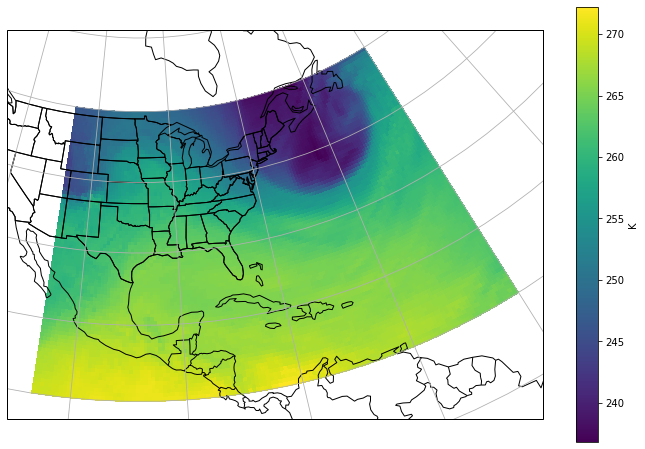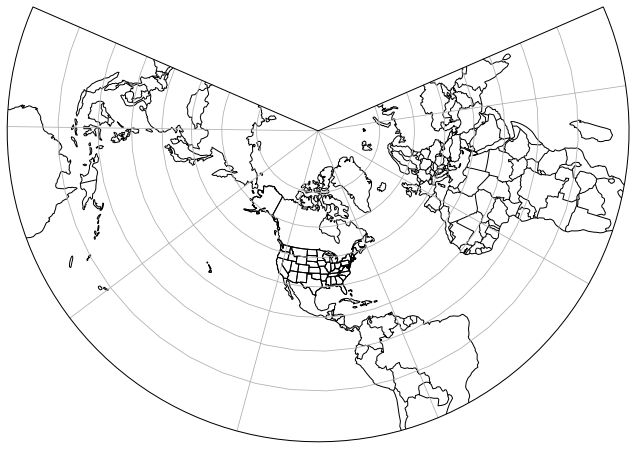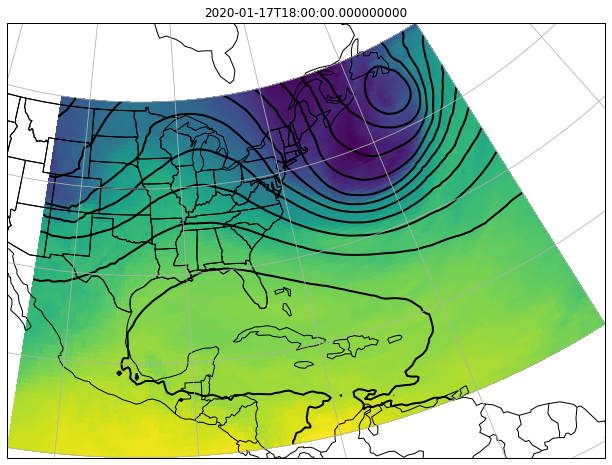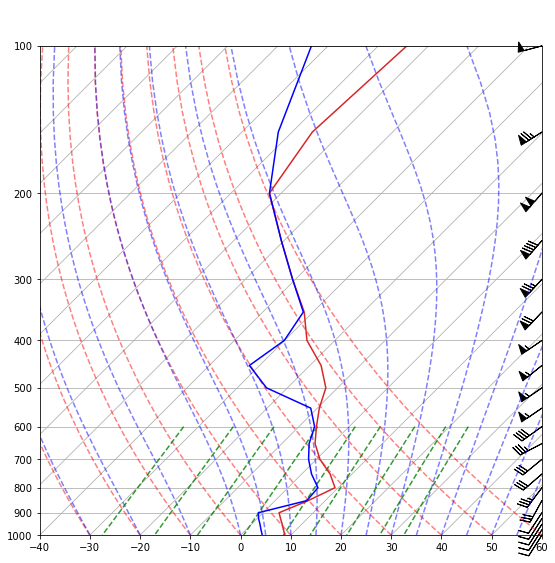#### Unidata Python Workshop#### Questions¶

1. What is the netCDF Subset Service (NCSS)?
2. How can I use Siphon to make an NCSS request?
3. How do I plot gridded fields using CartoPy?

### 1. What is NCSS?¶

In :
# Resolve the latest GFS dataset
import metpy
from siphon.catalog import TDSCatalog

# Set up access via NCSS
gfs_catalog = ('http://thredds.ucar.edu/thredds/catalog/grib/NCEP/GFS/'
'Global_0p5deg/catalog.xml?dataset=grib/NCEP/GFS/Global_0p5deg/Best')
cat = TDSCatalog(gfs_catalog)
ncss = cat.datasets.subset()


We can see what variables are available from ncss as well:

In :
ncss.variables

Out:
{'5-Wave_Geopotential_Height_isobaric',
'Absolute_vorticity_isobaric',
'Albedo_surface_Mixed_intervals_Average',
'Apparent_temperature_height_above_ground',
'Best_4_layer_Lifted_Index_surface',
'Categorical_Freezing_Rain_surface',
'Categorical_Freezing_Rain_surface_Mixed_intervals_Average',
'Categorical_Ice_Pellets_surface',
'Categorical_Ice_Pellets_surface_Mixed_intervals_Average',
'Categorical_Rain_surface',
'Categorical_Rain_surface_Mixed_intervals_Average',
'Categorical_Snow_surface',
'Categorical_Snow_surface_Mixed_intervals_Average',
'Cloud_Work_Function_entire_atmosphere_single_layer_Mixed_intervals_Average',
'Cloud_mixing_ratio_hybrid',
'Cloud_mixing_ratio_isobaric',
'Cloud_water_entire_atmosphere_single_layer',
'Composite_reflectivity_entire_atmosphere',
'Convective_Precipitation_Rate_surface_Mixed_intervals_Average',
'Convective_available_potential_energy_pressure_difference_layer',
'Convective_available_potential_energy_surface',
'Convective_inhibition_pressure_difference_layer',
'Convective_inhibition_surface',
'Convective_precipitation_rate_surface',
'Convective_precipitation_surface_Mixed_intervals_Accumulation',
'Dewpoint_temperature_height_above_ground',
'Field_Capacity_surface',
'Geopotential_height_highest_tropospheric_freezing',
'Geopotential_height_isobaric',
'Geopotential_height_maximum_wind',
'Geopotential_height_potential_vorticity_surface',
'Geopotential_height_surface',
'Geopotential_height_tropopause',
'Geopotential_height_zeroDegC_isotherm',
'Graupel_snow_pellets_hybrid',
'Graupel_snow_pellets_isobaric',
'Ground_Heat_Flux_surface_Mixed_intervals_Average',
'Haines_index_surface',
'ICAO_Standard_Atmosphere_Reference_Height_maximum_wind',
'ICAO_Standard_Atmosphere_Reference_Height_tropopause',
'Ice_cover_surface',
'Ice_growth_rate_altitude_above_msl',
'Ice_water_mixing_ratio_hybrid',
'Ice_water_mixing_ratio_isobaric',
'Icing_severity_isobaric',
'Land-sea_coverage_nearest_neighbor_land1sea0_surface',
'Land_cover_0__sea_1__land_surface',
'Latent_heat_net_flux_surface_Mixed_intervals_Average',
'MSLP_Eta_model_reduction_msl',
'Maximum_temperature_height_above_ground_Mixed_intervals_Maximum',
'Meridional_Flux_of_Gravity_Wave_Stress_surface_Mixed_intervals_Average',
'Minimum_temperature_height_above_ground_Mixed_intervals_Minimum',
'Momentum_flux_u-component_surface_Mixed_intervals_Average',
'Momentum_flux_v-component_surface_Mixed_intervals_Average',
'Ozone_Mixing_Ratio_isobaric',
'Per_cent_frozen_precipitation_surface',
'Planetary_Boundary_Layer_Height_surface',
'Potential_Evaporation_Rate_surface',
'Potential_temperature_sigma',
'Precipitable_water_entire_atmosphere_single_layer',
'Precipitation_rate_surface',
'Precipitation_rate_surface_Mixed_intervals_Average',
'Pressure_convective_cloud_bottom',
'Pressure_convective_cloud_top',
'Pressure_height_above_ground',
'Pressure_high_cloud_bottom_Mixed_intervals_Average',
'Pressure_high_cloud_top_Mixed_intervals_Average',
'Pressure_low_cloud_bottom_Mixed_intervals_Average',
'Pressure_low_cloud_top_Mixed_intervals_Average',
'Pressure_maximum_wind',
'Pressure_middle_cloud_bottom_Mixed_intervals_Average',
'Pressure_middle_cloud_top_Mixed_intervals_Average',
'Pressure_of_level_from_which_parcel_was_lifted_pressure_difference_layer',
'Pressure_potential_vorticity_surface',
'Pressure_reduced_to_MSL_msl',
'Pressure_surface',
'Pressure_tropopause',
'Rain_mixing_ratio_hybrid',
'Rain_mixing_ratio_isobaric',
'Relative_humidity_entire_atmosphere_single_layer',
'Relative_humidity_height_above_ground',
'Relative_humidity_highest_tropospheric_freezing',
'Relative_humidity_isobaric',
'Relative_humidity_pressure_difference_layer',
'Relative_humidity_sigma',
'Relative_humidity_sigma_layer',
'Relative_humidity_zeroDegC_isotherm',
'Sensible_heat_net_flux_surface_Mixed_intervals_Average',
'Snow_depth_surface',
'Snow_mixing_ratio_hybrid',
'Snow_mixing_ratio_isobaric',
'Soil_temperature_depth_below_surface_layer',
'Specific_humidity_height_above_ground',
'Specific_humidity_pressure_difference_layer',
'Storm_relative_helicity_height_above_ground_layer',
'Sunshine_Duration_surface',
'Surface_Lifted_Index_surface',
'Temperature_altitude_above_msl',
'Temperature_height_above_ground',
'Temperature_high_cloud_top_Mixed_intervals_Average',
'Temperature_isobaric',
'Temperature_low_cloud_top_Mixed_intervals_Average',
'Temperature_maximum_wind',
'Temperature_middle_cloud_top_Mixed_intervals_Average',
'Temperature_potential_vorticity_surface',
'Temperature_pressure_difference_layer',
'Temperature_sigma',
'Temperature_surface',
'Temperature_tropopause',
'Total_cloud_cover_boundary_layer_cloud_Mixed_intervals_Average',
'Total_cloud_cover_convective_cloud',
'Total_cloud_cover_entire_atmosphere_Mixed_intervals_Average',
'Total_cloud_cover_high_cloud_Mixed_intervals_Average',
'Total_cloud_cover_isobaric',
'Total_cloud_cover_low_cloud_Mixed_intervals_Average',
'Total_cloud_cover_middle_cloud_Mixed_intervals_Average',
'Total_ozone_entire_atmosphere_single_layer',
'Total_precipitation_surface_Mixed_intervals_Accumulation',
'U-Component_Storm_Motion_height_above_ground_layer',
'V-Component_Storm_Motion_height_above_ground_layer',
'Ventilation_Rate_planetary_boundary',
'Vertical_Speed_Shear_potential_vorticity_surface',
'Vertical_Speed_Shear_tropopause',
'Vertical_velocity_geometric_isobaric',
'Vertical_velocity_pressure_isobaric',
'Vertical_velocity_pressure_sigma',
'Visibility_surface',
'Volumetric_Soil_Moisture_Content_depth_below_surface_layer',
'Water_equivalent_of_accumulated_snow_depth_surface',
'Water_runoff_surface_Mixed_intervals_Accumulation',
'Wilting_Point_surface',
'Wind_speed_gust_surface',
'Zonal_Flux_of_Gravity_Wave_Stress_surface_Mixed_intervals_Average',
'u-component_of_wind_altitude_above_msl',
'u-component_of_wind_height_above_ground',
'u-component_of_wind_isobaric',
'u-component_of_wind_maximum_wind',
'u-component_of_wind_planetary_boundary',
'u-component_of_wind_potential_vorticity_surface',
'u-component_of_wind_pressure_difference_layer',
'u-component_of_wind_sigma',
'u-component_of_wind_tropopause',
'v-component_of_wind_altitude_above_msl',
'v-component_of_wind_height_above_ground',
'v-component_of_wind_isobaric',
'v-component_of_wind_maximum_wind',
'v-component_of_wind_planetary_boundary',
'v-component_of_wind_potential_vorticity_surface',
'v-component_of_wind_pressure_difference_layer',
'v-component_of_wind_sigma',
'v-component_of_wind_tropopause'}

From here, we can build a query to ask for the data we want from the server.

In :
from datetime import datetime, timedelta

# Create a new NCSS query
query = ncss.query()

# Request data in netCDF format
query.accept('netcdf')

query.variables('Temperature_isobaric')

# Ask for the 500 hPa surface
query.vertical_level(50000)

# Set the time range of data we want
now = datetime.utcnow()
query.time_range(now, now + timedelta(days=1))

# Set the spatial limits
query.lonlat_box(west=-110, east=-45, north=50, south=10)

# get the data!
data = ncss.get_data(query)


### 2. Making sense of netCDF¶

In :
data

Out:
<class 'netCDF4._netCDF4.Dataset'>
root group (NETCDF3_CLASSIC data model, file format NETCDF3):
Originating_or_generating_Center: US National Weather Service, National Centres for Environmental Prediction (NCEP)
Originating_or_generating_Subcenter: 0
GRIB_table_version: 2,1
Type_of_generating_process: Forecast
Analysis_or_forecast_generating_process_identifier_defined_by_originating_centre: Analysis from GFS (Global Forecast System)
Conventions: CF-1.6
history: Read using CDM IOSP GribCollection v3
featureType: GRID
History: Translated to CF-1.0 Conventions by Netcdf-Java CDM (CFGridWriter2)
Original Dataset = /data/ldm/pub/native/grid/NCEP/GFS/Global_0p5deg/GFS-Global_0p5deg.ncx3; Translation Date = 2020-01-17T15:15:14.524Z
geospatial_lat_min: 10.0
geospatial_lat_max: 50.0
geospatial_lon_min: -110.0
geospatial_lon_max: -45.0
dimensions(sizes): time(8), isobaric6(1), lat(81), lon(131)
variables(dimensions): float32 Temperature_isobaric(time,isobaric6,lat,lon), float64 reftime(time), float64 time(time), float32 isobaric6(isobaric6), float32 lat(lat), float32 lon(lon), int32 LatLon_Projection()
groups: 

We can use a library called XArray to make working with this a little simpler

In :
from xarray.backends import NetCDF4DataStore
import xarray as xr

# We need the datastore so that we can open the existing netcdf dataset we downloaded
ds = xr.open_dataset(NetCDF4DataStore(data))

In :
var = ds.metpy.parse_cf('Temperature_isobaric')
var

Out:
<xarray.DataArray 'Temperature_isobaric' (time: 8, isobaric6: 1, lat: 81, lon: 131)>
array([[[[246.11313, ..., 247.91313],
...,
[269.71313, ..., 264.71313]]],

...,

[[[247.01167, ..., 242.91167],
...,
[269.31168, ..., 264.51166]]]], dtype=float32)
Coordinates:
reftime    (time) datetime64[ns] ...
* time       (time) datetime64[ns] 2020-01-17T18:00:00 ... 2020-01-18T15:00:00
* isobaric6  (isobaric6) float32 50000.0
* lat        (lat) float32 50.0 49.5 49.0 48.5 48.0 ... 11.5 11.0 10.5 10.0
* lon        (lon) float32 250.0 250.5 251.0 251.5 ... 313.5 314.0 314.5 315.0
crs        object Projection: latitude_longitude
Attributes:
long_name:                      Temperature @ Isobaric surface
units:                          K
abbreviation:                   TMP
grid_mapping:                   LatLon_Projection
Grib_Variable_Id:               VAR_0-0-0_L100
Grib2_Parameter:                [0 0 0]
Grib2_Parameter_Discipline:     Meteorological products
Grib2_Parameter_Category:       Temperature
Grib2_Parameter_Name:           Temperature
Grib2_Level_Type:               100
Grib2_Level_Desc:               Isobaric surface
Grib2_Generating_Process_Type:  Forecast

XArray handles parsing things like dates, times, latitude, and longitude for us

In :
latitude = var.metpy.y
longitude = var.metpy.x


### Visualize the grid¶

In :
%matplotlib inline
import cartopy.crs as ccrs
import cartopy.feature as cfeature
import matplotlib.pyplot as plt

# GFS uses lon/lat grid
data_projection = ccrs.PlateCarree()

# Make it easy to change what time step we look at
t_step = 0

fig = plt.figure(figsize=(12, 8))
ax = fig.add_subplot(1, 1, 1, projection=ccrs.LambertConformal())
mesh = ax.pcolormesh(longitude, latitude, var[t_step].squeeze(),
transform=data_projection, zorder=0)

# add some common geographic features

ax.gridlines()

cax = fig.colorbar(mesh)
cax.set_label(var.attrs['units'])EXERCISE:
• Extend the plot above by plotting contours of 500 hPa geopotential heights
• Add a title to the plot with the correct time
In :
# Set up an NCSS query from thredds using siphon
query = ncss.query()

#
# SET UP QUERY
#

#ncss.get_data(query)

data_projection = ccrs.PlateCarree()

# Plot using CartoPy and Matplotlib
fig = plt.figure(figsize=(12, 8))
ax = fig.add_subplot(1, 1, 1, projection=ccrs.LambertConformal())

#
#

# add some common geographic features

ax.gridlines()

Out:
<cartopy.mpl.gridliner.Gridliner at 0x7f6f35022050>SOLUTION
In :
# %load solutions/map.py

# Cell content replaced by load magic replacement.
import numpy as np

# Set up an NCSS query from thredds using siphon
query = ncss.query()
query.accept('netcdf4')
query.variables('Temperature_isobaric', 'Geopotential_height_isobaric')
query.vertical_level(50000)
now = datetime.utcnow()
query.time_range(now, now + timedelta(days=1))
query.lonlat_box(west=-110, east=-45, north=50, south=10)

data = ncss.get_data(query)
ds = xr.open_dataset(NetCDF4DataStore(data))

temp_var = ds.metpy.parse_cf('Temperature_isobaric')
height_var = ds.metpy.parse_cf('Geopotential_height_isobaric')
longitude = temp_var.metpy.x
latitude = temp_var.metpy.y
time_index = 0

# Plot using CartoPy and Matplotlib
fig = plt.figure(figsize=(12, 8))
ax = fig.add_subplot(1, 1, 1, projection=ccrs.LambertConformal())

contours = np.arange(5000, 6000, 80)
ax.pcolormesh(longitude, latitude, temp_var[time_index].squeeze(),
transform=data_projection, zorder=0)
ax.contour(longitude, latitude, height_var[time_index].squeeze(), contours, colors='k',
transform=data_projection, linewidths=2, zorder=1)
ax.set_title(temp_var.metpy.time[time_index].values)

# add some common geographic features

ax.gridlines()

Out:
<cartopy.mpl.gridliner.Gridliner at 0x7f6f34eff310>### 4. NCSS Point Request¶

We can also request data for a specfic lon/lat point, across vertical coordinates or times.

In :
cat = TDSCatalog('http://thredds.ucar.edu/thredds/catalog/grib/NCEP/GFS/'
'Global_0p5deg/catalog.xml?dataset=grib/NCEP/GFS/Global_0p5deg/Best')
ncss = cat.datasets.subset()

point_query = ncss.query()
point_query.time(datetime.utcnow())
point_query.accept('netcdf4')
point_query.variables('Temperature_isobaric', 'Relative_humidity_isobaric')
point_query.variables('u-component_of_wind_isobaric', 'v-component_of_wind_isobaric')
point_query.lonlat_point(-101.877, 33.583)

# get the data! Unfortunately, xarray does not quite like what comes out of thredds
point_data = ncss.get_data(point_query)


Skew-T diagrams typical use specific units. First, let's assign units to the variables we requested:

In :
from metpy.units import units
import numpy as np

# get netCDF variables
pressure = point_data.variables["isobaric"]
dname_temp = point_data.variables["Temperature_isobaric"].dimensions
lev_temp = point_data.variables[dname_temp]
temp = point_data.variables["Temperature_isobaric"]
u_cmp = point_data.variables["u-component_of_wind_isobaric"]
v_cmp = point_data.variables["v-component_of_wind_isobaric"]
relh = point_data.variables["Relative_humidity_isobaric"]

# Need to put units on the left to assure things work properly with masked arrays
p = units(pressure.units) * pressure[:].squeeze()
T = units(temp.units) * temp[:].squeeze()
u = units(u_cmp.units) * u_cmp[:].squeeze()
v = units(v_cmp.units) * v_cmp[:].squeeze()
relh = units('percent') * relh[:].squeeze()

# Due to a different number of vertical levels find where they are common
_, _, common_ind = np.intersect1d(pressure, lev_temp, return_indices=True)
T = T[common_ind]


We also need to calculate dewpoint from our relative humidity data:

In :
import metpy.calc as mpcalc

Td = mpcalc.dewpoint_rh(T, relh)

/home/travis/miniconda/envs/unidata/lib/python3.7/site-packages/metpy/xarray.py:655: MetpyDeprecationWarning: The dewpoint_rh function was deprecated in version 0.12. This function has been renamed dewpoint_from_relative_humidity.
return func(*args, **kwargs)
/home/travis/miniconda/envs/unidata/lib/python3.7/site-packages/pint/numpy_func.py:289: RuntimeWarning: divide by zero encountered in log
result_magnitude = func(*stripped_args, **stripped_kwargs)


Now, let's change those units to what we typically see used in Skew-T diagrams. We use ito to do this in-place rather than manually reassigning to the same variable.

In :
p.ito(units.millibar)
T.ito(units.degC)
Td.ito(units.degC)
u.ito(units.knot)
v.ito(units.knot)

In :
from metpy.plots import SkewT

# Create a new figure. The dimensions here give a good aspect ratio
fig = plt.figure(figsize=(9, 9))
skew = SkewT(fig, rotation=45)

# Plot the data using normal plotting functions, in this case using
# log scaling in Y, as dictated by the typical meteorological plot
skew.plot(p, T, 'tab:red')
skew.plot(p, Td, 'blue')
skew.plot_barbs(p, u, v)
skew.ax.set_ylim(1000, 100)
skew.ax.set_xlim(-40, 60)

# Add the relevant special lines

<matplotlib.collections.LineCollection at 0x7f6f37745c10>